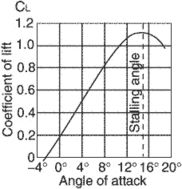# coefficient of lift

Also found in: Acronyms.

## coefficient of lift (CL)A form in which aerodynamic data is presented. A lift coefficient is dependent upon the angle of attack, shape of the wing section and plan form, condition of the wing surface, Mach number, and Reynolds number. Since most other factors are constant, CL values are plotted against the angle of attack. The value of CL rises up to the stalling angle, where it falls off rapidly. Lift coefficient (CL) = Lift (L)/Dynamic Pressure (q) × Wing Area (S) or CL = L/qS, or 2L/ρ V2S.
References in periodicals archive ?
Total Lift Force = Airplane Weight + Tail Down Force 2150 pounds = 2000 pounds + 150 pounds Table 1 Lift Force = Coefficient of Lift * Air Density / 2 * Wing Surface Area * [Airspeed.sup.2] Table 2 CG LOCATION AND SPIN RECOVER
[S.sub.l,pl] Shape coefficient of lift 12 t Wave period 1 [t] Average wave period 1 [t.sub.r] Return time 1.14 u Water velocity at the substratum 3 U Maximal water velocity 9 x Breaking force 22 [Chi] Mean breaking force 22 [Alpha] Coefficient of proportionality 2 [Beta.sub.d] Velocity exponent of drag 7 [Beta.sub.l] Velocity exponent of lift 12 [Zeta] Density of water 7 [Tau] Interval of time 1 [Tau.sub.i] Interval of time 1.17 [Tau.sub.tot] Total time 1.10 [Sigma] Standard deviation of breaking force 22
where [Beta.sub.l], is the velocity exponent of lift and [S.sub.l,pl] is the shape coefficient of lift, defined using the object's planform area.
The resulting yaw brings the left wing forward, decreasing [alpha] and thus bringing it back to the front side of the coefficient of lift curve and increasing lift.
Aircraft manufacturers, NASA and the de-ice equipment purveyors have done enough research to demonstrate even relatively benign ice accretion--if there is such a concept when it comes to icing--on a wing's leading can reduce the maximum coefficient of lift by as much as 30 percent, according to Dennis Newton's excellent treatise on the subject, Severe Weather Flying.
There are several useful and well-known kinds of performance curves out there in "Theoryland." Among the first to which we're introduced is the one depicting the steady rise of a dimensionless number called the coefficient of lift with increasing angle of attack, increasing at somewhere around 0.1 per degree up to anywhere between 1.0 and 1.8, and leveling off at this thing called [CL.sub.max], represented as the top-most point of the red line in the chart at right labeled "Lift vs.
As the angle between the wing chord and the relative wind is increased for a given airfoil, the coefficient of lift increases.

Site: Follow: Share:
Open / Close